# High School Geometry - Congruent Triangles

## Introduction

• Two polygons are congruent if and only if their vertices can be paired up so that their corresponding parts are congruent.
• The idea of congruent figures must come from "same size and same shape."
• Another thing to consider is we can pair up or match up corresponding parts of one figure to another same figure.
• Pairing up or matching up the vertices of congruent polygons is a one-to-one correspondence.
• Study the figures below. Note that it is important that the figures must fit exactly to do the proper pairing of vertices.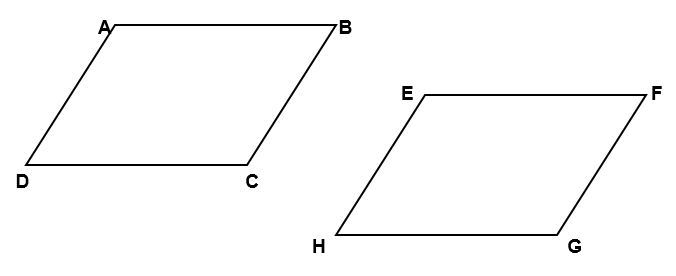$A↔E$ (A corresponds to E)

$B↔F$ (B corresponds to F)

$C↔G$ (C corresponds to G)

$D↔H$ (D corresponds to H)

Thus, we can say that $\square ABCD↔\square EFGH$. This leads us to have the following corresponding parts (angles and sides):

$\angle A$ and $\angle E$, $\angle B$ and $\angle F$, $\angle C$ and $\angle G$, and $\angle H$

$\overline{)AB}$ and $\overline{)EF}$$\overline{)BC}$ and $\overline{)FG}$$\overline{)CD}$ and $\overline{)GH}$$\overline{)AD}$ and $\overline{)EH}$

• In the case of triangles, consider the triangles $△ABC$ and $△XYZ$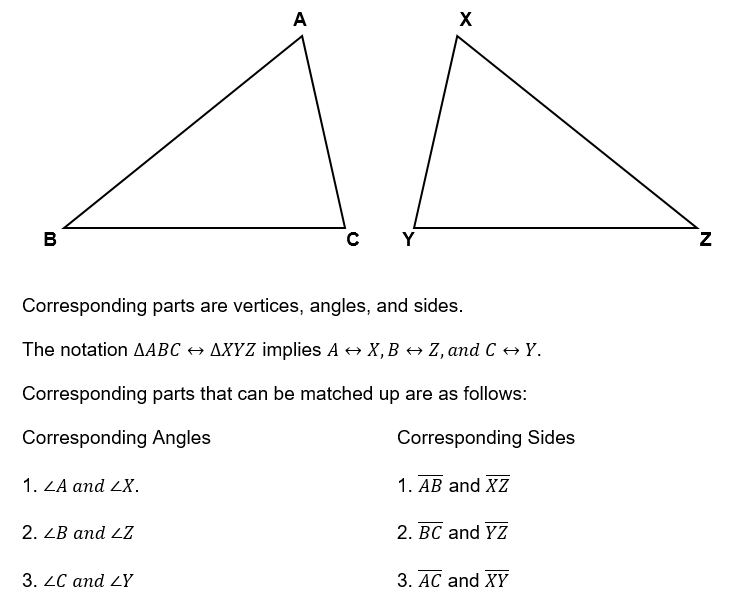• For congruent triangles, corresponding sides and corresponding angles are congruent or CPCTC.

## Geometric Proofs and Congruence Postulates

• To validate that two triangles are congruent, one must apply the congruence postulates and theorem in writing geometric proofs.
• The process of logic has been essential to many professionals. In the same manner, proving in geometry requires skills in logic and reasoning to write proofs as solutions to a problem. This procedure can be a deductive form or an inductive form.
• Suppose that we wish to prove that $△DAE\cong △CBE$ with $\overline{)AD}\cong \overline{)BC}$ and $\overline{)AD}\parallel \overline{)BC}$. Using this example, we have the if-then statement: "If $\overline{)AD}\cong \overline{)BC}$ and $\overline{)AD}\parallel \overline{)BC}$, then $△DAE\cong △CBE$."
• The flow of conducting a formal proof is done by writing the given conditions in the statement column and by writing the definitions, properties, theorems, and postulates in the reasons column.
• Since the measurement of sides and angles are positive real numbers, then the properties of real numbers are applicable to them.
• Below are the properties of congruence and equality that will serve as a guide:

1. Reflexive Property:

For angles, we have: $m\angle A=m\angle A$ or $\angle A\cong \angle A$

For segments, we have: $XY=XY$ or $\overline{)XY}\cong \overline{)XY}$

2. Symmetric Property:

If $\angle A=\angle B$, then $\angle B=\angle A$

If $\angle A\cong \angle B$, then $\angle B\cong \angle A$.

If $AB=XY$, then $XY=AB$.

3. Transitive Property:

If $\angle A=\angle B$ and $\angle B=\angle C$, then $\angle A=\angle C$.

If $\angle A\cong \angle B$ and $\angle B\cong \angle C$, then $\angle A\cong \angle C$.

• Proofs can be done in various ways such as:

1. Two-Column Proof

2. Paragraph Form

3. Use of a Flow Chart

TRIANGLE CONGRUENCE POSTULATES AND THEOREM

• SSS (Side-Side-Side) Postulate - If the three sides of one triangle are congruent to the corresponding three sides of another triangle, then the two triangles are congruent.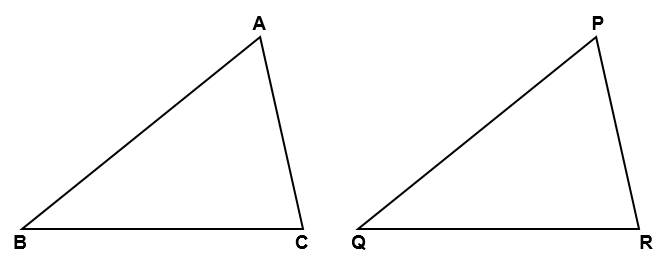If and $\overline{)BC}\cong \overline{)QR}$, then $△ABC\cong △PQR$ by SSS Postulate.

• SAS (Side-Angle-Side) Postulate - If two sides and an included angle of one triangle are congruent to the corresponding two sides and an included angle of another triangle, then the two triangles are congruent.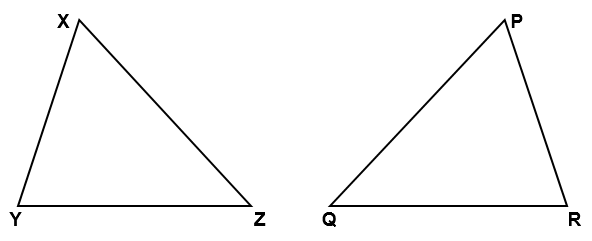If and $\angle Y\cong \angle R$, then $△XYZ\cong △PRQ$ by SAS Postulate.

• ASA (Angle-Side-Angle) Postulate - If the two angles and an included side of one triangle are congruent to the corresponding two angles and an included side of another triangle, then the triangles are congruent.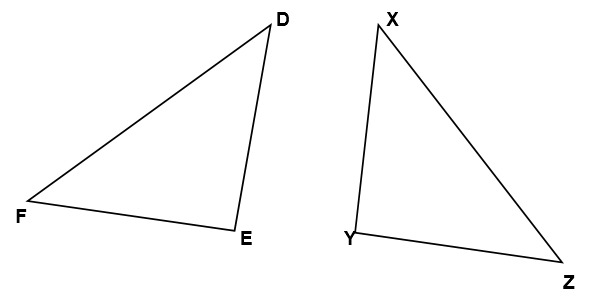If and $\angle E\cong \angle Y$, then $△DEF\cong △XYZ$ by ASA Postulate.

• SAA (Side-Angle-Angle) Theorem - If two angles and an excluded side of one triangle are congruent to the corresponding two angles and an excluded side of another triangle, then the two triangles are congruent.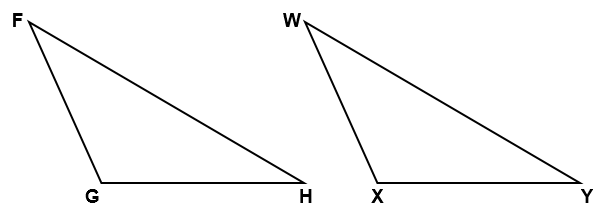If and $\overline{)FH}\cong \overline{)WY}$, then $△FGH\cong △WXY$ by SAA Theorem.

Right Triangle Congruence

• LL (Leg-Leg) Theorem - If the two legs of one right triangle are congruent to the corresponding legs of another right triangle, then the two right triangles are congruent.
• LA (Leg-Angle) Theorem - If a leg and an acute angle of one right triangle are congruent to the corresponding leg and an acute angle of another right triangle, then the two right triangles are congruent.
• HA (Hypotenuse-Angle) Theorem - If the hypotenuse and an acute angle of one right triangle are congruent to the corresponding hypotenuse and an acute angle of another right triangle, then the two right triangles are congruent.
• HL (Hypotenuse-Leg) Theorem - If the hypotenuse and leg of one right triangle are congruent to the corresponding hypotenuse and leg of another triangle, then the two right triangles are congruent.

Isosceles Triangle Theorem

• If two sides of a triangle are congruent, then the angles opposite these sides are congruent.
• Converse: If two angles of a triangle are congruent, then the sides opposite these angles are congruent.

## Solved Examples

Example 1. Refer to the proof below.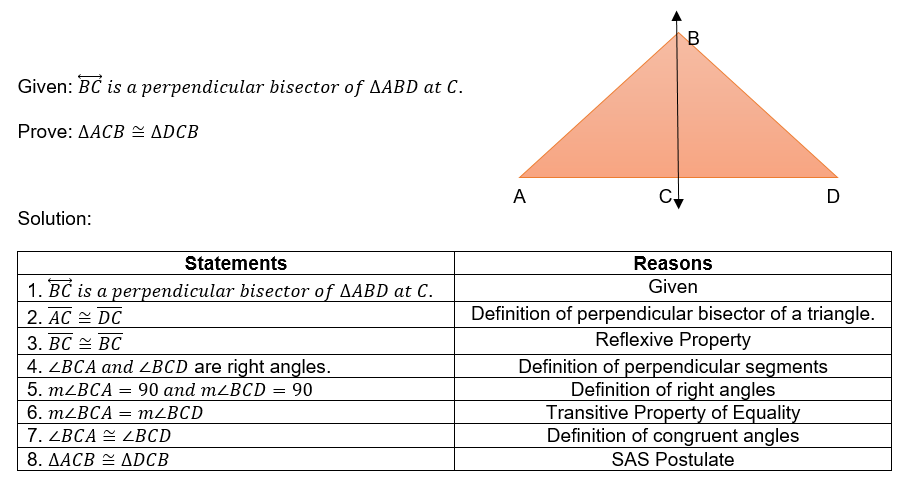Example 2. Study the proof below.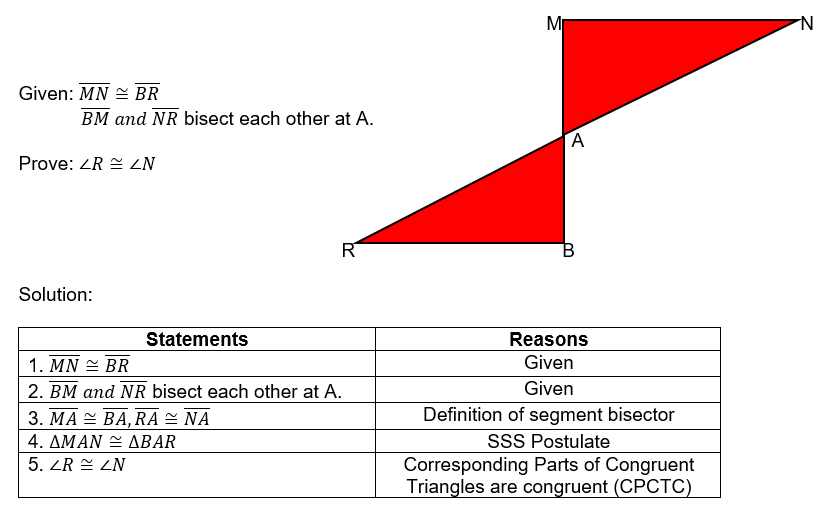Example 3. $△ABC\cong △RTU$ by SAS Postulate. If and $RU=2x-6$, find the measures of angles and sides of the two congruent triangles.

Solution:

$\angle A=\angle R$

$3x+15=x+75$

$2x=60\to x=30$

$⇒\angle A=\angle R=105°$

$AB=RT$

$2x+15=3x-15$

$x=15$

$⇒AB=RT=75$

$AC=RU$

$x+4=2x-6$

$x=10$

$⇒AC=RU=14$

## Cheat Sheet

• Two triangles are congruent if and only if their corresponding angles and corresponding sides are congruent.
• Congruent polygons must have the same size and same shape.
• Two triangles can be proven using the following tests for congruence:

1. SSS (Side-Side-Side) Postulate

2. SAS (Side-Angle-Side) Postulate

3. ASA (Angle-Side-Angle) Postulate

4. SAA (Side-Angle-Angle) Theorem

• To prove that two right triangles are congruent, we can use the following theorems:

1. LL (Leg-Leg) Theorem

2. LA (Leg-Angle) Theorem

3. HA (Hypotenuse-Angle) Theorem

4. HL (Hypotenuse-Leg) Theorem

• Any two polygons are congruent if their corresponding angles are congruent and their corresponding sides are congruent.

## Blunder Areas

• Congruent polygons are not the same as the concept of similar polygons.
• Polygons that have the same shape but different sizes are not congruent.
• In naming congruent polygons or triangles, always be mindful of the order of their vertices. The order of vertices of one figure should be the same as that of the other figure.
• Matching up vertices, angles, and sides of two polygons or triangles must be fitted exactly to show the congruence of the two geometric figures.
• One-to-one correspondence of corresponding parts is necessary to determine the corresponding congruent parts.
• In the case of circles, these figures can be considered congruent if and only if their radii have equal measures.
• In the case of solving problems without given diagrams or figures of congruent triangles, it is helpful to draw a reasonably accurate sketch to visualize the corresponding congruent parts.